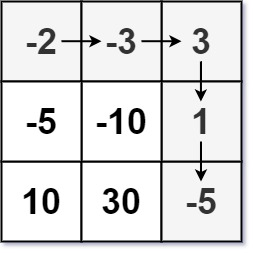304 North Cardinal St.
Dorchester Center, MA 02124

# Dungeon Game LeetCode Solution

## Problem – Dungeon Game LeetCode Solution

The demons had captured the princess and imprisoned her in the bottom-right corner of a `dungeon`. The `dungeon` consists of `m x n` rooms laid out in a 2D grid. Our valiant knight was initially positioned in the top-left room and must fight his way through `dungeon` to rescue the princess.

The knight has an initial health point represented by a positive integer. If at any point his health point drops to `0` or below, he dies immediately.

Some of the rooms are guarded by demons (represented by negative integers), so the knight loses health upon entering these rooms; other rooms are either empty (represented as 0) or contain magic orbs that increase the knight’s health (represented by positive integers).

To reach the princess as quickly as possible, the knight decides to move only rightward or downward in each step.

Return the knight’s minimum initial health so that he can rescue the princess.

Note that any room can contain threats or power-ups, even the first room the knight enters and the bottom-right room where the princess is imprisoned.

Example 1:``````Input: dungeon = [[-2,-3,3],[-5,-10,1],[10,30,-5]]
Output: 7
Explanation: The initial health of the knight must be at least 7 if he follows the optimal path: RIGHT-> RIGHT -> DOWN -> DOWN.
``````

Example 2:

``````Input: dungeon = []
Output: 1
``````

Constraints:

• `m == dungeon.length`
• `n == dungeon[i].length`
• `1 <= m, n <= 200`
• `-1000 <= dungeon[i][j] <= 1000`

## Dungeon Game LeetCode Solution in C++

``````class Solution {
public:
int calculateMinimumHP(vector<vector<int> > &dungeon) {
int M = dungeon.size();
int N = dungeon.size();
// hp[i][j] represents the min hp needed at position (i, j)
// Add dummy row and column at bottom and right side
vector<vector<int> > hp(M + 1, vector<int>(N + 1, INT_MAX));
hp[M][N - 1] = 1;
hp[M - 1][N] = 1;
for (int i = M - 1; i >= 0; i--) {
for (int j = N - 1; j >= 0; j--) {
int need = min(hp[i + 1][j], hp[i][j + 1]) - dungeon[i][j];
hp[i][j] = need <= 0 ? 1 : need;
}
}
return hp;
}
};
``````

## Dungeon Game LeetCode Solution in Python

``````class Solution:
def calculateMinimumHP(self, dungeon):
m, n = len(dungeon), len(dungeon)
dp = [[float("inf")]*(n+1) for _ in range(m+1)]
dp[m-1][n], dp[m][n-1] = 1, 1

for i in range(m-1,-1,-1):
for j in range(n-1,-1,-1):
dp[i][j] = max(min(dp[i+1][j],dp[i][j+1])-dungeon[i][j],1)

return dp
``````

## Dungeon Game LeetCode Solution in Java

``````public int calculateMinimumHP(int[][] dungeon) {
if (dungeon == null || dungeon.length == 0 || dungeon.length == 0) return 0;

int m = dungeon.length;
int n = dungeon.length;

int[][] health = new int[m][n];

health[m - 1][n - 1] = Math.max(1 - dungeon[m - 1][n - 1], 1);

for (int i = m - 2; i >= 0; i--) {
health[i][n - 1] = Math.max(health[i + 1][n - 1] - dungeon[i][n - 1], 1);
}

for (int j = n - 2; j >= 0; j--) {
health[m - 1][j] = Math.max(health[m - 1][j + 1] - dungeon[m - 1][j], 1);
}

for (int i = m - 2; i >= 0; i--) {
for (int j = n - 2; j >= 0; j--) {
int down = Math.max(health[i + 1][j] - dungeon[i][j], 1);
int right = Math.max(health[i][j + 1] - dungeon[i][j], 1);
health[i][j] = Math.min(right, down);
}
}

return health;
}
``````
##### Dungeon Game LeetCode Solution Review:

In our experience, we suggest you solve this Dungeon Game LeetCode Solution and gain some new skills from Professionals completely free and we assure you will be worth it.

If you are stuck anywhere between any coding problem, just visit Queslers to get the Dungeon Game LeetCode Solution

Find on Leetcode

##### Conclusion:

I hope this Dungeon Game LeetCode Solution would be useful for you to learn something new from this problem. If it helped you then don’t forget to bookmark our site for more Coding Solutions.

This Problem is intended for audiences of all experiences who are interested in learning about Data Science in a business context; there are no prerequisites.

Keep Learning!

More Coding Solutions >>

LeetCode Solutions

Hacker Rank Solutions

CodeChef Solutions